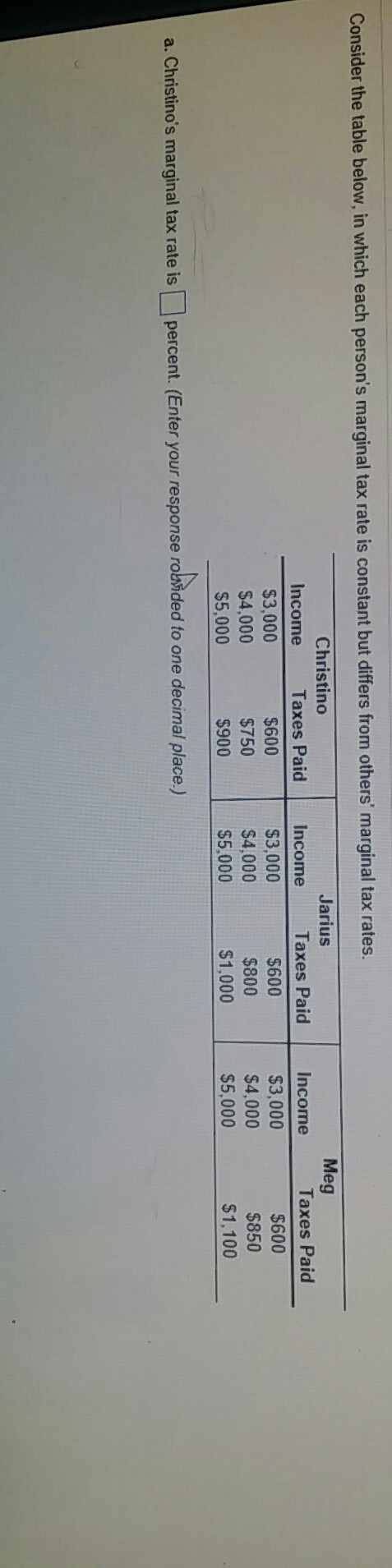# Question & Answer: Consider the table below, in which each person's marginal tax rate is constant but differs from others marginal tax rate…..Consider the table below, in which each person’s marginal tax rate is constant but differs from others marginal tax rates. a. Christino marginal tax rate is percent. (Enter your response rounded to one decimal place.)

Don't use plagiarized sources. Get Your Custom Essay on
Question & Answer: Consider the table below, in which each person's marginal tax rate is constant but differs from others marginal tax rate…..
GET AN ESSAY WRITTEN FOR YOU FROM AS LOW AS \$13/PAGE

Christonio’s Marginal Tax rate is 15%

Marginal Tax rate is calculated as under

Marginal Tax rate

=( changes in tax paid / changes in income) *100

Marginal Tax rate when income changes from 3000 to 4000

=((750-600) / (4000-3000) ) *100

=(150/1000)*100

=15%

Now we repeat the same calculation for when income changes from 4000 to 5000

Marginal Tax rate when income changes from 4000 to 5000

=((900-750) / (5000-4000) ) *100

=(150/1000)*100

=15%

So here we can say that marginal tax rate for Christoni is 15%